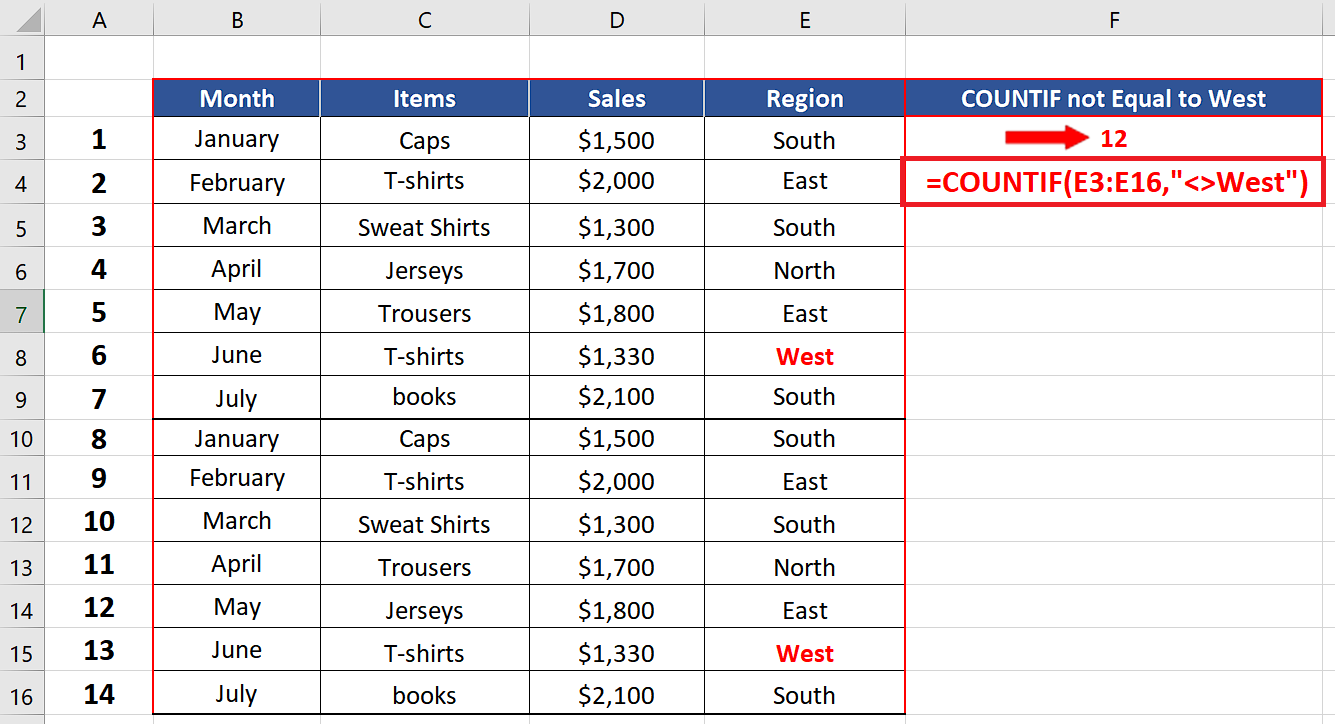# How to use COUNTIF to count when cell is not equal to a specific value in Excel

In this tutorial we will learn how we can use Excel’s built-in “COUNTIF” function to count the number of cells which are not equal to a specific value.

The best way to understand how COUNTIF works is to first understand the structure of the function and the parameters it requires.

## Structure of COUNTIF

The standard syntax of COUNTIF function in excel is shown below;

= COUNTIF(range,criteria)

• range (where to look in for something)

A range can be a single column with multiple rows or it could consist of multiple columns and multiple rows. For example “A1:B10”. This will tell the formula to search through two columns A & B till the 10th row.

• criteria (what to look for)

Criteria will be a special logical test which will depend upon the situation and the nature of values that we are looking for. In our example we are looking for all cells which are not equal to the text West so we will use a special logical condition in the formula which will tell Excel to count only those cells which are not equal to the text West.

## Implementing the Formula on an Actual Data Set

Let’s take an example data set and find out the number of sales which are from regions other than West. So, let’s consider the data set shown above.

Let’s see how we can use Excel’s built-in formulas to find a solution to our problem by following these steps;When we are working with data sets to analyze them in Excel, often there is a requirement to count those cells which do not meet a specific criterion or not equal to a specific value. Excel provides us with a function to find out the number of such cells in your dataset.

### Step 1 – Create a formula using COUNTIF– Choose a suitable cell where you wish to implement the formula.
– Use the following formula in that cell and press enter key.
=COUNTIF(E2:E14,”<>West”)
– The desired result will be displayed in this cell as soon as you will press the enter key as shown in the picture above.

So by using COUNTIF with a special condition to count the sales from all regions other than West in column E we can find out that 12 sales are completed by regions other than West.

## Breakdown of the Logical Condition used inside the formula

We used the formula =COUNTIF(E2:E14,”<>West”)

Let’s see how we told Excel where to look and what to look for.

• The first parameter E2:E14 tells Excel that the data range in which we are going to look for something is in column E and from row 2 to row 14 only.
• The second parameter was “<>West”. This represents a logical condition i.e., Not Equal to West. So, when we use this combination in the condition parameter it tells Excel to count the cells in the range E2:E14 that are not equal to West. When Excel finds any cell meeting the criteria, the counter is increased. Our data set had only 2 cells out of 14 in that range which had West so the result was 12 as desired.